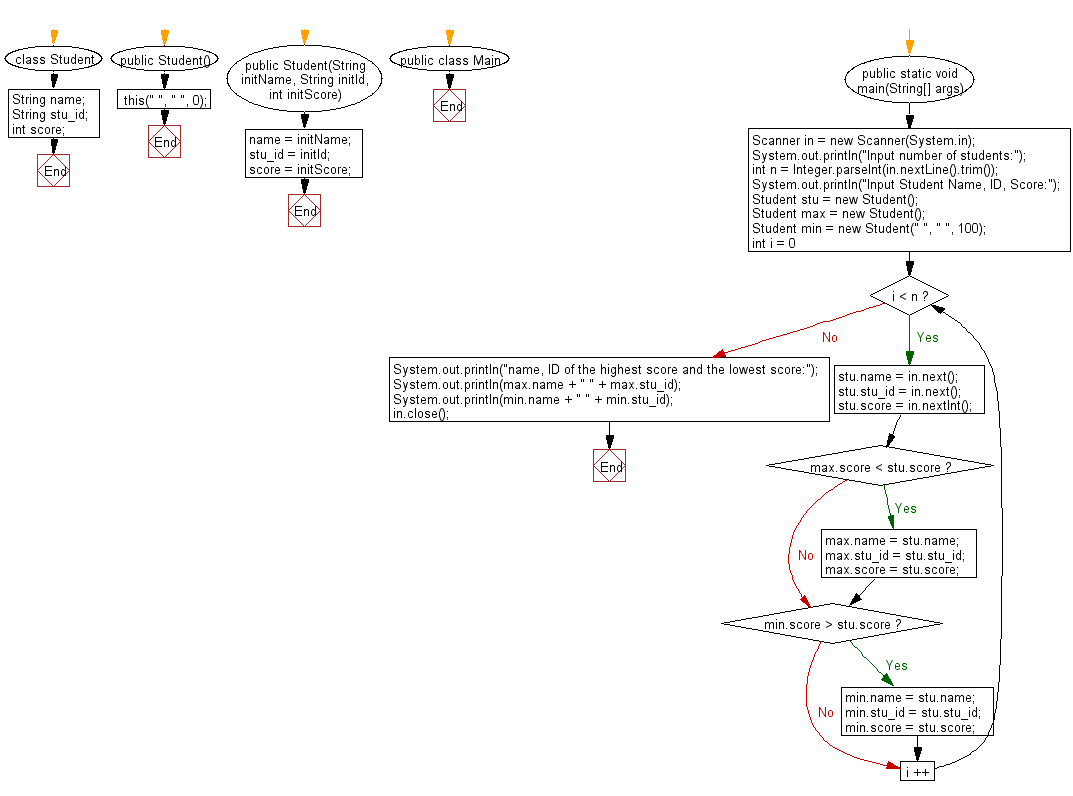# Java Exercises: Accepts students name, id, and marks and display the highest score and the lowest score

## Java Basic: Exercise-245 with Solution

Write a Java program which accepts students name, id, and marks and display the highest score and the lowest score.

The student name and id are all strings of no more than 10 characters. The score is an integer between 0 and 100.

Sample Solution:

Java Code:

``````import java.util.Scanner;

class Student {
String name;
String stu_id;
int score;
public Student() {
this(" ", " ", 0);
}
public Student(String initName, String initId, int initScore) {
name = initName;
stu_id = initId;
score = initScore;
}
}

public class Main {
public static void main(String[] args) {
Scanner in = new Scanner(System.in);
System.out.println("Input number of students:");
int n = Integer.parseInt(in.nextLine().trim());
System.out.println("Input Student Name, ID, Score:");
Student stu = new Student();
Student max = new Student();
Student min = new Student(" ", " ", 100);
for (int i = 0; i < n; i ++) {
stu.name = in.next();
stu.stu_id = in.next();
stu.score = in.nextInt();
if (max.score < stu.score) {
max.name = stu.name;
max.stu_id = stu.stu_id;
max.score = stu.score;
}
if (min.score > stu.score) {
min.name = stu.name;
min.stu_id = stu.stu_id;
min.score = stu.score;
}
}
System.out.println("name, ID of the highest score and the lowest score:");
System.out.println(max.name + " " + max.stu_id);
System.out.println(min.name + " " + min.stu_id);
in.close();
}
}
```
```

Sample Output:

```Input number of students:
3
Input Student Name, ID, Score:
Devid v1 72
Peter v2 68
Johnson v3 85
name, ID of the highest score and the lowest score:
Johnson v3
Peter v2
```

Pictorial Presentation:Flowchart:Java Code Editor:

What is the difficulty level of this exercise?

﻿

## Java: Tips of the Day

Parsing dates:

```import java.io.*;
import java.util.*;
import java.text.*;

String s = "2001/09/23 14:39";

SimpleDateFormat formatter = new SimpleDateFormat ("yyyy/MM/dd H:mm");
Date d = formatter.parse(s, new ParsePosition(0));
```